﻿ Flows of Vectors Induction Conductor Structures in Electronic Systems

### Flows of Vectors Induction Conductor Structures in Electronic Systems

V.G. Kudrya

Digital Technologies

## Flows of Vectors Induction Conductor Structures in Electronic Systems

Department of Information Systems and Networks, Odessa National Academy of Food Technologies, Odessa, Ukraine

### Abstract

The research algorithms for analysis of electromagnetic communication state conductor structures of electronic systems and components. Held effective selection and improvement of existing methods for calculating the electromagnetic parameters interconnect speed digital and analog electronic means. Algorithms based on calculating the flow of the electric and magnetic induction of surfaces are specified construction of monolithic integrated circuits.

• V.G. Kudrya. Flows of Vectors Induction Conductor Structures in Electronic Systems. Digital Technologies. Vol. 1, No. 1, 2015, pp 52-60. http://pubs.sciepub.com/dt/1/1/10
• Kudrya, V.G.. "Flows of Vectors Induction Conductor Structures in Electronic Systems." Digital Technologies 1.1 (2015): 52-60.
• Kudrya, V. (2015). Flows of Vectors Induction Conductor Structures in Electronic Systems. Digital Technologies, 1(1), 52-60.
• Kudrya, V.G.. "Flows of Vectors Induction Conductor Structures in Electronic Systems." Digital Technologies 1, no. 1 (2015): 52-60.

 Import into BibTeX Import into EndNote Import into RefMan Import into RefWorks

1
Prev Next

### 1. Introduction

Modern features new technology is widely used electromagnetic processes and phenomena. Development of monolithic integrated circuits, nanotechnology , electrical and metal smelting, electrolysis, induction cleaning semiconductors, electrical minerals, electrical protection against corrosion of underground structures - not a complete list of areas where analysis of electromagnetic processes plays a leading role.

Regardless, in different forms and purposes all modern electrical and electronic devices have the same part - electromagnetic system which is used to convert energy. This system is: a set of metal wires with currents and insulation that prevents leaks current conduction; magnetic conductors (steel parts) used for amplification and concentration of magnetic flux; screens of various shapes that prevent unauthorized flows of energy and ensure electromagnetic compatibility. The properties of such systems depends not only on the circuit implementation of their functions, but also on the distribution of volume, surface and linear the electromagnetic field of real design.

The fundamental work  on research dimension of space in the new orthogonal coordinate systems have enhanced the class of problems for which analytical solution can be obtained. However, their use is limited to the requirement of symmetry and coincidence surfaces: surface distribution environments with the coordinate surface. However, their use is limited to the requirement of symmetry and coincidence surfaces - surface distribution environments with the coordinate surface, or - with its stereographic projection .

However, for systems with electromagnetic parts of complex shape and characteristics of non-linear environments field could not count because of the lack of effective methods of calculating and computing facilities. Most electrical problems solved experimentally, and for the calculation of electromagnetic fields rather than strict mathematical methods using semi-empirical methods based on a synthesis of experience, analogies and engineering intuition.

The emergence of highly efficient computer systems has opened up new opportunities for automation of the design of new technology and including computing, various means of information and computer technology, radio or television reception, mobile gadgets and components that have a monolithic integrated circuits. However, the level of physical and mathematical modeling currently does not have time for practice use of various natural phenomena, not only in complex technological systems, as well as their individual components. The level of integration of monolithic integrated circuits continuously increasing in line with Moore's rule with the transition to nanotechnology, spintronics and photonics. In other words, the problem of updating or developing new physical and mathematical models describing electromagnetic processes objects of new technology is quite relevant.

The purpose of this study is an attempt to improve the isolation and analysis of electromagnetic effective methods of communication conditions conductor structures of electronic systems and components. These algorithms must agree difficulty describing their temporal and capacitive load , on the other hand - meet the criteria of adequacy of the facility design .

### 2. Common Methods of Electromagnetic Condition

Modern CAD electrical and electronic devices require standard software for fast and accurate calculation of the electromagnetic field in the system any reasonable geometry. To create such programs should develop universal and effective mathematical methods for the analysis of electromagnetic processes in inhomogeneous and nonlinear environments with free form surfaces media division. The complexity of forms designs electrical devices preclude the use of analytical calculations, so with the advent of high-speed computer systems were extensively developed numerical methods. However, it soon turned out that the use of numerical methods for the calculation of electromagnetic processes faces serious difficulties. The amount of memory and computing machinery required for the calculation with preset accuracy, expressed astronomical numbers far beyond the capabilities of modern super computer systems. Out of this crisis seek to improve computational methods and increasing computing power, but not enough attention is paid to the possibility of yet another improvement. To explain what was going on, we consider the course of calculating electromagnetic process. The task of electromagnetic fields formulated on the basis of the fundamental laws of electrodynamics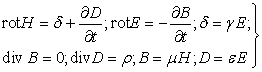(1)

where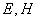(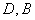)- intensity vector (induction) electric and magnetic fields- a source of the electromagnetic field, current density vector (scalar charge density);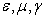- material parameters of the environment.

Vectors on the surface distribution of environments e and i satisfy the boundary conditions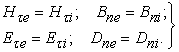(2)

where indices τ and n tangential and normal projection vectors corresponding to the limit distribution environments.

Equations (1) and (2) theoretical model called electromagnetic process. The theoretical model is supplemented by information on the geometry of the boundaries media division, their material parameters, intensity spatial distribution of sources, includes a latent form all the information about electromagnetic process.

Having a number of assumptions and simplifications, reflecting the specificity of this class of electromagnetic fields on the basis of theoretical models, build a mathematical model. Thus, to calculate the fields for which the displacement current densityin equation (1) is much smaller compared to the density of conduction currents δ, injected quasi-stationary condition for which the source of the magnetic field are considered only of real current carriers. However, the condition of quasi-stationary does not deny the change vector field over time at all, as is done in the analysis of electrostatic fields fixed charges and fixed magnetic fields generated by DC. According to equations (1) is a product of the electric component of the electromagnetic field alternating magnetic field at the time. Without limiting generalization assume that electromagnetic values change over time for sinusoidal law, and material parameters assume constant values that the vast majority of it is quite possible.

The mathematical model of a boundary value problem is obtained by introducing the potentials A and φ relationships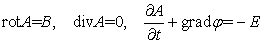(3)

and the resulting system (1) in piecewise homogeneous medium to the Poisson equation for the potential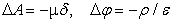(4)

or more complex equations in a heterogeneous environment.

Transforming the boundary conditions (2) according to the specificity of the field, come to the boundary value problem for differential equations in partial derivatives. As proved that the staged thus boundary value problem has a unique solution, build a mathematical model at this stage usually finish, considering any additional information redundant. These mathematical models emerged long before computers and numerical methods and focused on the application only accurate analytical methods. It is not interested in the amount of information processing needed for numerical solution of the problem, and the ability to automate this processing. This, perhaps, due to the desire to build a mathematical model using only the necessary minimum of information derived from theoretical models. This meant that all the properties of the electromagnetic process obtained by exact analytical solution of the boundary problem. When the opportunity numerical study of electromagnetic processes, reasoning underlying the mathematical model in the form of analytical boundary problem, were forgotten. Major efforts are not directed at modernizing the type of mathematical model and to improve class computational methods suitable for her research.

But between the analytical and numerical solutions is a significant difference. The exact analytical solution is a creative, heuristic that is resistant to automate the process of converting information without her loss and distortion. As a result, the analytical solutions, all information contained in the boundary-value problem is transformed into a compact, foreseeable form that is presented in the form of a limited aggregate functions studied. The nature of the analytical solution contains information about many of these processes, different values of the parameters.

Unlike analytical, numerical solution of the boundary value problem - a formalized process using standard methods and allows automation. Numeric conversion information related to its distortion, as suggests digitization. When replacing the difference-differential equations, functions and continuous boundary surfaces are represented as piecewise continuous, so that background information is distorted. Iterative process and rounding errors distort information in the process of transformation. The more complex the task, the more information there is distortion in the process of obtaining numerical solution, the less accurate result of the decision. Trying to achieve the desired accuracy decreasing grid spacing leads to an increase in the number of unknown values of unknown function. This dramatically increases the amount of computation, and hence the accumulation of errors. Numerical solution containing incomplete, distorted information about a specific process.

### 3. System Construction Algorithm

To determine a reasonable compromise between algorithmic complexity and functional generalization of mathematical models advisable to perform differentiation processes in narrow classes with common features in geometry, properties of the environment and character in the time. Under these conditions within each class generally accepted scheme for calculating the electromagnetic process can be divided into three stages. First, a substantiation and choice of assumptions (axioms). Second, the construction of mathematical models by analytical partial conversion of the information contained in the theoretical model (based on accepted assumptions) into a form suitable for a particular task. Usually it comes down to the formulation of the boundary value problem, which is reduced to a system of differential equations in partial derivatives, or integral equations. Thirdly, obtaining numerical algorithm for computing process, in other words, the numerical transformation information form for specific answers to request that interested developers design object.

The weak point of the design scheme is the third phase - the computational process of solving the problem. Here there are all the difficulties and errors. The conclusion refuse to convert information only numerical methods and accordingly change the design scheme. Most of the changes necessary to try to do exactly analytically, thus reducing the amount of change, remaining at a fraction of numerical methods, which are the source of accumulation of errors.

Analytical transformation should be subjected to the most common piece of information inherent in all objective of this class. Transforming it once, the result can be used to build new mathematical model in which to numerical processing will only selective information reflecting the specifics of a particular problem.

From a theoretical model for obtaining information about the properties of the integrated process, together with previous data use it to build a new mathematical model. The more the precise information on the properties of electromagnetic process will be put into a mathematical model, the fewer properties will be determined by means of numerical transformations. Introduction of additional information into the mathematical model narrows the class of functions, which looks for solutions, reduces the amount of computation and creates distortions limitation of incidental information in the calculation.

To analyze the complex system to use the maximum in advance information found on the desired solution, but not least, hiding its use getting super-generalized solution. Calculation of electromagnetic process should be carried out in several stages, alternating analytical transformation, where they can be done with numeric, leaving the car just routine of processing that meets individual needs a certain stage of the calculation.

The cause distortions introduced during sampling show at the famous example of the Laplace approximation of differential difference equation. Setting grid spacing h and replacing the derivatives at the point of potential φ0 ratios finite increments, are: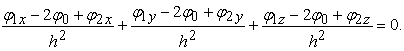Hence the importance of this potential, resulting in the end because of the boundary conditions is defined as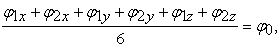(5)

where - value potential φ in the center of each of the six faces of the cube cell environment; φ0 - potential values at the center of the cube. On the other hand, the basic property of harmonic functions is equal to the average value of capacity building in the center of the field areas that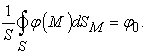(6)

Obviously, the expressions (5) and (6) coincide only in a uniform field, when potential - a linear function of coordinates. Thus, the difference approximation of the Laplace operator distorts the basic property of unknown function. A similar picture is the approximation and other difference-differential operators. Within the method of finite differences is distortion can be reduced only by reducing grid spacing h. But it sharply increased the number of unknown values of potential, even more dramatically increase the amount of credit and the accumulation of errors by limiting word length "computer" numbers. The attempt to minimize distortion of information that makes sampling leads to increased distortion arising in the numerical conversion. The situation further worsened when calculating unshielded field. The outer limit of the field is not defined, and the calculation method of finite differences appears methodological error due to artificially limit the study area field.

### 4. Analytical Numerical Algorithms

Consider a design scheme that uses analytical information transformation at intermediate stages results. It is known  that in an infinite vacuum electromagnetic process for a given source distribution can be calculated accurately by the formulas(7)

where c - speed of light in vacuum;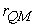- distance between points of observation Q and source M. In this case, the problem does not arise - potentials and their derivatives are expressed as quadratures through the source and can be calculated in the space of any required accuracy. All the complexity of calculation conditioned by the heterogeneity of environment, since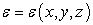,and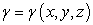the formulas (7) illegal.

However, the rejection of "a comprehensive" solution with the transition to the solution selected in "working" areas that are characterized by homogeneous material parameters allows you to get the desired result. This approach was proposed by introducing fictitious sources that simulate heterogeneity . Like Method of images, these secondary sources are on the verge of distribution irregularities. They replace the heterogeneous environment in uniform in the location of primary sources.

This feature allows you to bring the system of equations (1), which describes the field in a heterogeneous environment, to a system that describes the equivalent field in vacuum by introducing secondary currents and charges in places where changing characteristics of the environment. This problem is reduced to determining the coordinates of the location and intensity of secondary sources, which fundamentally changes the mathematical model. This path leads to a system of Fredholm integral equations of the second order with respect to the densities of secondary sources. Secondary sources are usually localized in space, so the number of unknown dramatically decreases that improves the accuracy of the final solution. Solving integral equations and finding the distribution of secondary sources through formulmy (7) easy to identify a field anywhere in the workspace Q.

This model is interesting is the fact that it can enter additional information about the integral properties the sought field: current, voltage, the flow of the electric and magnetic induction, chain concept current and voltage, etc. To this end, these values should be brought to the form of conditions to be met by the distribution of secondary sources. A new mathematical model compared to the old model, in the form of a boundary value problem, has reduced the number of unknowns, providing the necessary accuracy, about two or three order, but the calculation of electromagnetic field system, even in this formulation of the problem is difficult, since it leads to the necessity of solving the liabilities systems of equations with unknowns 103-104. This forced to go through further specialization mathematical model. The order of the equation system can be reduced by narrowing the class of problems for which designed mathematical model. Thus, instead of a mathematical model to analyze a wide class of quasi-stationary fields occurring in technology, we can construct a series of models to analyze the various classes, such as static fields in heterogeneous environments in nonlinear media, quasi-stationary fields in ferromagnetic media, piecewise homogeneous environments, and so on. Expertise reduces the possibility of a mathematical model, but increases the accuracy of the calculation of fields for which the model is intended.

Calculation of electromagnetic process can also be facilitated by using a mathematical model building engineering considerations. Due to the specific morphology electronic device characteristics of the environment is sometimes possible to guess the basic structure the sought field and use this information to simplify the model. Obtained in this way additional information is approximate character and inevitably leads to coarsening of model and narrowing the class of problems that have quality solutions. However, if you guessed right about the correct use, you can simplify the model so that when the numerical solution of the problem significantly increase accuracy. Classic examples of such mathematical models can serve as a system of equations of the theory of electrical circuits and homogeneous lines. Such a model, for example, can be a complex mathematical model of the electromagnetic micro and nanotechnology process electronic circuit fed by current with high frequency. The chain is a connection of thin metal wires permanent cross section located on a dielectric plate, which shielded the top and bottom flat metal plates. When the flow of current in the wire electromagnetic field permeates all elements of the chain. The distribution of current and voltage in this circle is determined not only galvanic connections and connection circuit elements, but strong electromagnetic interactions between all its wires.

Analyzing the boundary conditions on the surface of the wire, easy to find that the electric field inside the wire nearly one-dimensional (normal to the axis of the wire vector component of the electric field is almost zero), in other words, almost all the current flows along the axis of the wire. On the surface of the dielectric wire, in contrast, electric induction vector is almost zero axial component of the tension that the bias current is directed normal to the lateral surface of the wire. Wire electromagnetic field deforms so that local areas near the surface electric field component becomes almost two-dimensional structure. Due to the smoothness of the potential functions of this structure will continue in some a layer thickness surrounding the wire. Thus, the electric field inside the wire one dimensional, in a thin layer surrounding the wire outside - two-dimensional, and the rest of the space - three-dimensional.

Small size diameter sections compared to wire length and the smoothness of the potential functions provide small changes in electrical parameters on the cut wires and allow them to replace the mean. Go to the average values in the simulation of electromagnetic processes allowed to enter the internal resistance of a wire and operate with a current, potential and linear density located on the wire charge. This reduced dimension equations and simplify the solution of the problem.

Keep in mind that discussed above micro- and nanotechnology electronic circuits surrounded by heterogeneous layered media - dielectrics and conductors. This greatly complicates the task. To simplify the solution without loss of accuracy in the mathematical model is introduced additional information about the structure of the electromagnetic field in a layered environment which is obtained analytically. For this wire chain replaced multiple series-connected radiating dipoles. The electromagnetic field excited wire is represented as the sum of fields created by each of the dipoles. In the stratified environment field excited dipole can be determined analytically by using Fourier transform.

The solution of integral equations of mathematical models can only approximate numerical methods. For this integration region is divided into the largest possible number of elements (contours, volume or surface) and, assuming known species density distribution of secondary sources within each element, replacing its approximate integral value in a finite amount. Thus the integral equation leads to a system of linear algebraic equations, the order is equal to the number of elements of the field of integration. Matrix coefficients calculated by integration of the kernel of the integral equation for each element region. Solving the system are approximate density distribution of secondary sources, then field and all his characteristics are calculated analytically by formulas (7). This method can be called a combined analysis, that analytical and numerical or qualitative.

### 5. Object-oriented Equations

Omitting the details of obtaining equations, which can be found in the works of O. Tozoni , let's algorithm analysis of high-frequency electromagnetic system electrical circuit, located on the the border of two dielectrics in multilayered environment. As noted, the structure of the algorithm in this case is the same as for the circuit in a homogeneous infinite medium. Differ only expressions for the conductivity layer planar field and the mean values of potentials.

The system of equations circuit with sinusoidal currents in a multilayer medium can be written as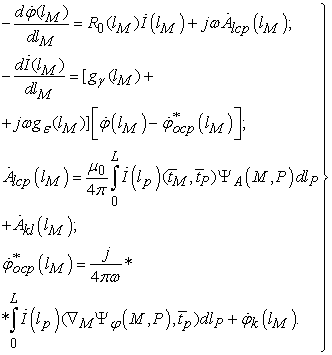(8)

where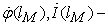current and potential distribution along the conductor, parametric coordinate length lM which varies from 0 to L; R0(lM) - the value of ohmic resistance of the conductor per unit of its length; ;; ω - cyclic frequency external power voltage connected to the points of the circle;; gγ(lM), gε(lM) - conductor and dielectric permittivity of the dielectric skin-deep equivalent of the conductor per unit of its length, determined in accordance leakage current and the displacement from the lateral surface;,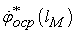- the average per unit length of the conductor mentioned projection vector and scalar potential on its axis. Their sources are, as a proper external source that is presented in the form of the first term curvilinear integral, and not their own extraneous sources, contributions are presented in accordance with the plugin,. The nuclei of integral-differential equations containing scalar product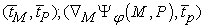that can interpret (8) as a scalar equation system. Functionsdepend on the structure of the medium surrounding the circuit and adjusted to the particular stage of modeling morphology chain. In particular, if the chain is in a homogeneous infinite medium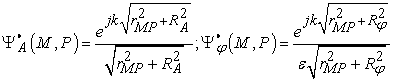(9)

The chain is a two-layer medium as specified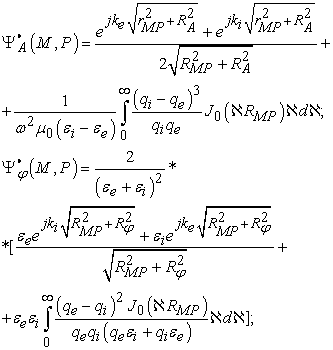(10)

For the location of a chain on insulating substrate with screen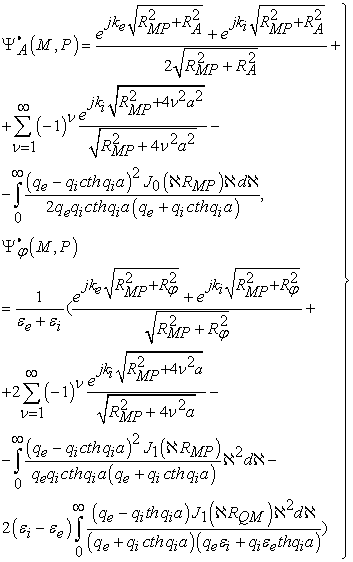(11)

The parameters, included in the system of equations (8) ... (11) for the circuit with the wire film having linear resistance, which does not depend on the length must satisfy the relation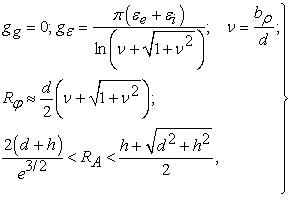(12)

where- small axis of the ellipse (section) which layer of field; 2d - width and 2h - thickness of the film section of wire. The calculations revealed the optimal value ν = νopt ≅ 0.1, providing minimize errors. Taking this value and assuming that h = 0.02d, are: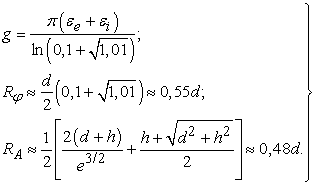(13)

It can be shown that the same form of equations chain will in any multilayer piecewise homogeneous medium.

Thus, the electromagnetic process in an electric circuit sinusoidal high frequency current, which is located in multilayer piecewise homogeneous medium, described by equations (8) ... (13). Functions,and settings g,,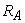included in this system depends on the structure of the medium surrounding the chain. As you can see, the specificity of solving systems of equations (8) depends on the morphological structure of the facility design. From the analysis of specific examples in the case of homogeneous structure chains can be found in . Integral equation is reduced to the system (8) differ only in dimension integrals, nuclear structure and expression of the right of integral equations. Integrals included in the equation are the potentials and their derivatives. Algorithms for numerical analysis of models with the same type of structure, consisting of: calculating the block coefficient matrix; vector calculation unit right side; block forming a system of equations and its solution; calculation unit vector fields and potentials; block calculation integral field parameters, including flow of the electric and magnetic induction.

The same type of structure equations of mathematical models and algorithms for their analysis automates the programming for calculation of electromagnetic systems. With the software package as building blocks for computing integrals expressing potentials and their various derivatives, can be the default program for analysis of various electromagnetic fields. The advantage of such mathematical models is the ease of automation. Within the class of problems methods of forming algorithms are independent of morphology. This means that for the same program without any adjustments it can solve a class of fields of different geometric patterns.

### 6. Qualitative Analysis Methods

The next step in the simplification model of electromagnetic system state leaders may be the use of qualitative methods of analysis for the initial choice of form analytical solution that would take into account the most essential properties of the field. One such method is based on the analogy of a plane and sphere of parallel fields (PP and SP) [2, 6].

In PP and SP fields flow per unit length (along the axis z, and radius r) can be found by using the tool of flow by avoiding computation of integrals operations. PP field called field that created charges (currents) located in unlimited of parallel wires. Let the direction of the wire coincides with the direction of z-axis of the Cartesian coordinate system. In each of the of parallel surfaces z = C picture field lines repeated. SP fields, like PP, created ray charges (currents), also located on the conductors (rays), but with a conical surface that begin at one point. Taking this point as the sphere of zero radius r = 0 in each of these spheres at r = C will have a recurrence pattern stereographic field lines.

Later also use the assumption that the potential fields of thin wires systems with finite radius can be considered roughly coincident with potentials fields threads (infinitely thin wires with zero radius), provided that the terms for their spatial definition is not possible to set coordinates internal points of the wires that obstruct the body of metal wire, considering these expressions are valid only outside the body wires.

As you know, the scalar potential φ space at any point (x, y) PP electrostatic field systems (n + 1) strands passing through Cartesian coordinates xk, yk, is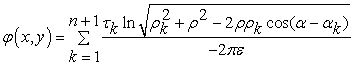(14)

where(15)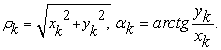(16)

By placing observation point on the surface of the m-th wire that has a radius аm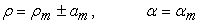(17)

we can get it potential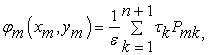(18)

where(19)

for each m = 1, 2, ..., n + 1 conductors.

If the result of calculations (18) does not depend on what the sign (+ or -) stayed in (17), it allows to justify the assumption of filaments overlap fields and fields of thin wires. Using (18) we form all potential difference(20)

where(21)

when changing from 1 m to n is taken into account vanishing of total charge of the system. Since the flux from the surface ‾D piece length lm is lmτm, solving the system of equations (20) with respect to τm, will have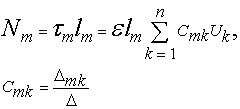(22)

where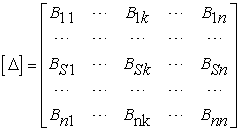(23)

When placing the wire in one plane (ym = yk = 0), or on the surface of a circular cylinder ρ = R we obtain according to Pmk:(24)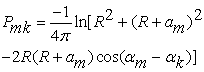(25)

For PP magnetic field consider that the flux of magnetic induction ‾B, which accounts for unit length, can be found as the difference vector potentials at points lying on two wires. Since the potential field n + 1 is a thread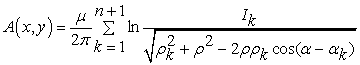(26)

on the surface of the m-th wire after substituting (17) into (26) we have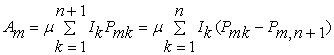(27)

where Pmk corresponds to the formula (19), and taking into account the zero total current system of currents: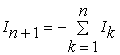(28)

The magnetic flux through the plane of length lm,, which relies on wire m and q, is equal to: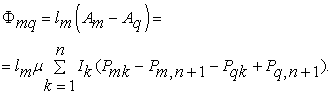(29)

In view of (21) flows through the neighboring induction vector by independent numbering contours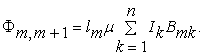(30)

Formula (22), (30) can be used not only for the system of cylindrical conductors, but also to calculate the flow induction vectors parallel narrow strip conductor. This should apply conformal transformation forms generatrix, as it is made in the derivation of (9) ... (13). To determine the flows in the system wires are crossed, it appears useful theory of SP fields. To consider SP fields are entered spherical coordinates r, θ, α.. They coordinate surfaces are: r = C - concentric spheres, θ=C - circular cones, α=C - half-plane. Instead, θ we consider the variable β, that changes in a specific way of scale polar coordinates preserving its direction.(31)

which together with the coordinates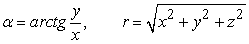(32)

specifies the location of points in space.

SP electric (magnetic) fields called field potentials which are independent of the coordinates r: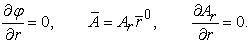(33)

The purpose of introducing the coordinates of (31) is a simplified form of recording two coordinate the Laplace equation for the SP field, which takes the form of two-dimensional Laplacian coincides with the Cartesian coordinate system. In this case, the potentials of φ and Аr satisfy the equations(34)

and the electric potential SP field potential recorded similar PP field. So PP field of charged parallel strands corresponds SP field of charged filaments rays with a common vertex. Location of each of the parallel branches posed coordinates ρк and αк. Define the location of each ray coordinates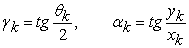(35)

Potential SP field system thread-rays will be written by analogy with (14)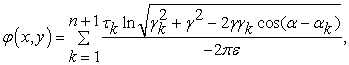(36)

where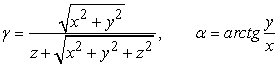(37)

and for the radial component of vector potential use expression (26)(38)

Still, one could argue that using potential flow calculation SP fields electric and magnetic induction can be done without conducting operations integration. We illustrate this for the flow of magnetic induction. Consider a filled annular sector 1234, Figure 1,a.Download asVeiw figureFigures index
Figure 1. Definition of magnetic flux ring sector (a); replacement of cone wire (b) as cylindrical (c)

The flow through the sector is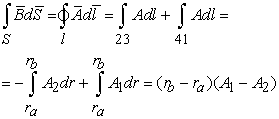(39)

Along the way (1,2) and (3,4) integrals(40)

performed because of orthogonality radial vector potential, the direction of which coincides with the direction of current I beam angle and direction of the path of integration that goes along arcs (1, 2) and (3.4), Fig. 1a. At intervals rays 23 and 14 values of the vector potential Ar is constant, because of SP field and therefore it is imposed in (39) under the integral sign. Thus, the flux of magnetic induction, which accounts for unit length in the direction of the radius vector ‾ r is the difference Ar potential at points through which the rays with current limiting annular sector.

In determining the flow field was used PP filaments potential field and limit the flow calculation was selected at a short distance from the filament. This distance equal to the radius of the wire. The same procedure for SP fields consists in choosing the threshold beam - outside the sector, which is located near the beam-thread with the current. To determine the potential value of φ and Ar on the surface of the conical wire Fig. 1b, it is necessary to put in (36) and (38) coordinates the conical surface of the conductor and its radius(41)

where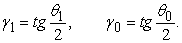(42)

To practice case where the wire has two sharp cones, Figure 1b, less interesting than the design of wires as thin curved cylinder, Figure 1c. Obviously, defined flow sector 1, 2, 3, 4 in field Figure 1b, there will be little different from the flow design Figure 1c for bent cylinders at small angles θ0, when executed ratio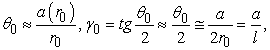(43)

where r0 the distance from the origin to the place where the radius of the cylindrical conductor "and" coincides with the intersection of the cone radius sphere having a radius r = r0. Obviously, if the length of the cylindrical wire from its beginning to the end sector is l, then 2r0=l and а = а (r0)- radius conical wire at a distance r0. Choice of size r0 at a given radius of a cylindrical wire "a" is related to issues such replacement cylinder by cone to flow in finding a place would not have a significant error. In this case, the "shortage" of magnetic flux at distance r0 > l offset by its excess at r0 < l.

In a similar manner calculated flux electric induction between beams that create an electric field. This cylindrical wire, Figure 1b consists of two insulated from each other halves (rays), which also applied voltage creates an electric field SP.

In most cases, under load mismatch and generator at the ends of two-wire line, charge distribution and current along the conductors looks exponentially-harmonic functions. In this case, to solve the problem, you can use a combination of the previous methods. In such communication structures having planar and spherical transverse electromagnetic waves (T-waves). These waves are captured with scalar and vector potentials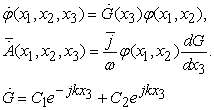(44)

where x1, x2 - orthogonal coordinates on the plane (sphere), and х3 - normal to the planes (fields) coordinate. Potential φ( x1, x2 ) is the Laplacian potential that is independent of frequency. Therefore, in practice the use of T-waves quite common: first, the transmission path of the T-wave is over вroadband (φ‘ω = 0), and secondly, the calculation of the potential static field is much easier to calculate electrodynamic potential. The name of transverse waves were that any plane (sphere) х3 electric and magnetic field lines are tangent, that no longitudinal component strengths Е3=0, Н3=0. Plate T-waves are directed parallel perfect conductor wires unlimited. Spherical T-wave radiation directed system of wires. Whereas, in addition to planar and spherical T-wave no other variables at the time of the electromagnetic fields that have the same structure of power lines, set charge distribution along the wire as(45)

where х3 = z for T-wave planar and х3 = r for a spherical T-wave.

Expressing constant С, С charge through at the beginning х3 = 0 and х3 = l the end of the line, you can (45) rewritten as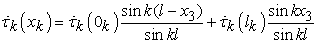(46)

Flux offrom a wire length l is(47)

Similarly we write the current distribution(48)

In accordance with (18), on the surface of the m-th wire in his points x3 х3 = 0 = 0 and х3 = l have the equation(49)

where to Pmk remains fair formula (19). From equation (20) form expressionsand(50)

where Вmk corresponds to the formula (21). Solving relative charge density equation (47) ... (50) we obtain vector flow induction system of parallel conductors with different potentials(51)

where Сmk determined by (22). For small values of the product klm can be considered a case of low-frequency T-wave and take into account that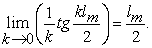(52)

Similarly, for the magnetic fields that permeate the plane length lm, which is based on wire m and q, is located the flow using the vector potential. Subject to (48) we obtain(53)

where to Bmk fair formula (21).

Thus, given algorithms allow calculation of flow vectors induction electromagnetic field of communicators monolithic integrated circuits, which are, conductor structures of both internal and external interconnections. The choice of algorithm depends on the ratio of the length of the electromagnetic waves generated by the device and its characteristic dimension.

### 7. The Conclusion

Solving problems of improving CAD Electronics brakes lack of adequate physical and mathematical models that combine simplicity with accuracy algorithmic models play a variety of factors, physical phenomena and processes that determine the characteristics of object design. The value of the mathematical model determined by the amount and quality of information obtained from a physical model. The more additional information about electromagnetic processes used to construct a mathematical model, the more effective appears its calculation algorithm . The more the information, the higher the quality and the better result of numerical solutions. The process of improving the mathematical model requires long creative effort, but satisfy the requirements dictated by modern software engineering with a vengeance will pay all costs. Along with the improvement of computer systems and numerical methods growing importance and theoretical studies, the main elements of which in relation to electronic communication elements presented in this paper. In particular formulated goals and objectives interconnect simulation of digital and analog electronic means. Depending on the level of idealization communicator structure analysis algorithms presented its electromagnetic condition that seamlessly integrates into the circuit model through electrodynamics parameters. Formulation of problems of electrodynamics in their calculation is based on the definition of induction flow vectors at specified surface conductor structures defined on structural and technological development phase electronic means. The latter allows you to perform signifies our strong synthesis morphology monolithic integrated circuits. Thus, the results will contribute to the development of the design of high-speed analog-to-digital means

### References

  Kudrya V.G. Modeling nanotechnology electronic means. Monograph [Text, Electronic resource] // Odessa National Academy of Food Technologies - Kherson. Oldie-Plus, 2013. - 780 p. - Access: http://nbuv.gov.ua/ (Ukr).In article  Knyaz A.I. Complex potentials of three-dimensional electric and magnetic fields: Monograph. - Kiev - Odessa: Vishcha School, 1981. 120 p. (Rus).In article  Knyaz A.I. Electrodynamics of information systems. - M .: Radio and Communications, 1994. 392 p. (Rus).In article  Bondarenko M.F. and others. Computer discrete mathematics. - Kharkov: “Smith”, 2004. 408 p. (Ukr).In article  Tozoni O.V. Method of secondary sources in electrical engineering. M.: Energia, 1975, 296 p. (Rus).In article  Knyaz A.I., Kudrya V.G. Electrodynamic model-based parametric video amplifier circuit design \ “Radiotechnics”, 1985, №6, - M.: Radio and communication. 1985. P. 87-88 (Rus).In article